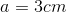# SAT II Math I : Right Triangles and Similar Triangles

## Example Questions

### Example Question #1 : Right Triangles And Similar Triangles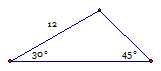Find the area of the triangle.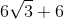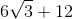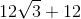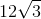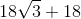Explanation: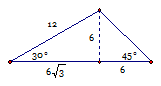Dropping the altitude creates two special right triangles as shown in the diagram.  Use the area formula of a triangle to get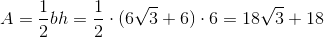### Example Question #2 : Right Triangles And Similar Triangles

Fire tower A ismiles due west of fire tower B.  Fire tower A sees a fire in the directiondegrees west of north.  Fire tower B sees the same fire in the directiondegrees east of north.  Which tower is closer to the fire and by how much?

Fire tower B; 0.24 miles

Fire tower A; 1.53 miles

The two fire towers are equidistant to the fire.

Fire tower A; 0.24 miles

Fire tower B; 1.29 miles

Fire tower B; 0.24 miles

Explanation: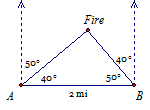First, realize that the angles given are from due north, which means you need to find the complements to find the interior angles of the triangle.  This triangle happens to be a right triangle, so the fast way to compute the distances is using trigonometry.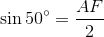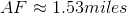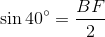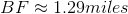Fire tower B is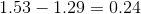miles closer to the fire.

### Example Question #1 : Right Triangles And Similar Triangles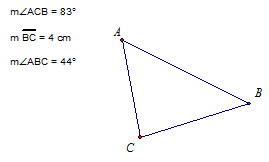Find the length of side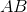.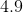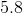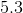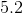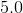Explanation:

In an angle-side-angle problem, Law of Sines will solve the triangle.

First find angle A: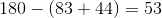Then use Law of Sines.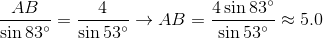### Example Question #4 : Right Triangles And Similar Triangles

What is the length of the leg of a right triangle whose hypotenuse is 5cm and other leg is 4cm?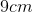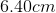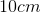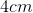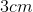Explanation: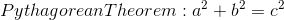One leg is 4cm and the hypotenuse is 5cm. Plug in 4 for one of the legs and 5 for the hypotenuse (c).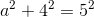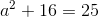Subtracting 16 from either side of the equation gives us: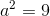The last step is to take the square root both sides resulting in: